• +91 9971497814
• info@interviewmaterial.com

# Chapter 3- Electrochemistry Interview Questions Answers

### Related Subjects

Question 1 :

How would you determine thestandard electrode potential of the systemMg2+ | Mg?

The standard electrode potentialof Mg2+ | Mg can be measured with respect to thestandard hydrogen electrode, represented by Pt(s),H2(g) (1 atm) | H+(aq)(1M).

A cell, consisting of Mg | MgSO4 (aq 1M) as the anode and the standard hydrogen electrode as the cathode, is set up.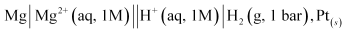Then, the emf of the cell ismeasured and this measured emf is the standard electrode potential of themagnesium electrode.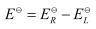Here,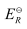for the standard hydrogenelectrode is zero.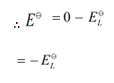Question 2 :

Can you store copper sulphatesolutions in a zinc pot?

Zinc is more reactive thancopper. Therefore, zinc candisplace copper from its salt solution. If copper sulphate solution is storedin a zinc pot, then zinc will displace copper from the copper sulphate solution.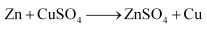Hence, copper sulphate solutioncannot be stored in a zinc pot.

Question 3 :

Consult the table of standardelectrode potentials and suggest three substances that can oxidise ferrous ions under suitable conditions.

Substancesthat are stronger oxidising agents than ferrous ions can oxidise ferrous ions.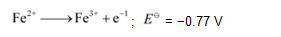This implies that the substances having higherreduction potentials than
+0.77 V can oxidise ferrous ions to ferric ions. Three substances that can doso are F2,Cl2,and O2.

Question 4 :

Calculate the potential ofhydrogen electrode in contact with a solution whose pH is 10.

Answer 4 :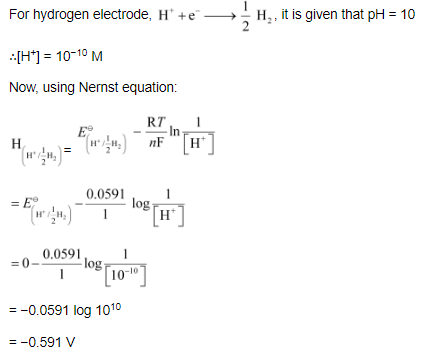Question 5 :

Calculate the emf of the cell inwhich the following reaction takes place: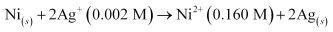Given that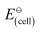= 1.05 V

Applying Nernst equation we have: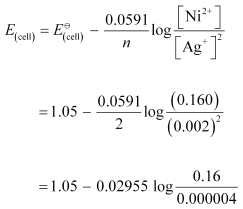= 1.05 − 0.02955 log 4 × 104

= 1.05 −0.02955 (log 10000 + log 4)

= 1.05 −0.02955 (4 + 0.6021)

= 0.914 V

Question 6 :

The cell in which the followingreactions occurs: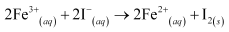has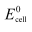= 0.236 V at 298 K.

Calculate the standard Gibbs energy and the equilibrium constant of the cell reaction.

Here, n =2,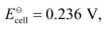T = 298 K

We know that: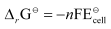= −2 × 96487 × 0.236

= −45541.864 J mol−1

= −45.54 kJ mol−1

Again,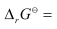−2.303RT log Kc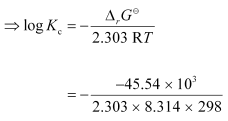= 7.981

Kc =Antilog (7.981)

= 9.57 × 107

Question 7 :

Why does the conductivity of asolution decrease with dilution?

The conductivity of a solution isthe conductance of ionspresent in a unit volume of the solution. The number of ions (responsible forcarrying current) decreases when the solution is diluted. As a result, theconductivity of a solution decreases with dilution.

Question 8 : Suggest a way to determine the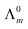value of water.

Answer 8 : Applying Kohlrausch’s law of independent migration ofions, thevalue of water can be determined as follows: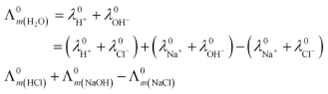Hence, by knowing thevalues of HCl, NaOH, and NaCl, thevalue of water can be determined.

Question 9 :

The molar conductivity of 0.025mol L−1 methanoic acid is

46.1 Scm2 mol−1.

Calculate its degree of dissociation and dissociation constant. Given λ (H_+)

= 349.6 S cm_2 mol_−1 and λ _°(HCOO−) = 54.6 S cm_2 mol

C =0.025 mol L−1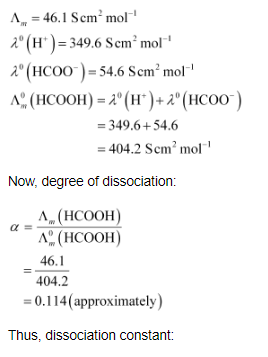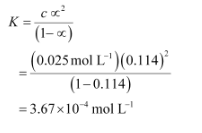Question 10 :

If a current of 0.5 ampere flowsthrough a metallic wire for 2 hours, then how many electrons would flow throughthe wire?

= 0.5 A

t = 2 hours = 2 × 60 × 60 s = 7200 s

Thus, Q = It

= 0.5 A ×7200 s

= 3600 C

Weknow that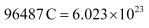number of electrons.

Then,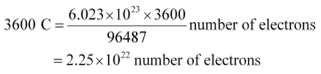Hence,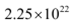number of electrons will flowthrough the wire.

Todays Deals### Chapter 3- Electrochemistry Contributorskrishan

Name:
Email:

# Latest News# 9000 interview questions in different categories